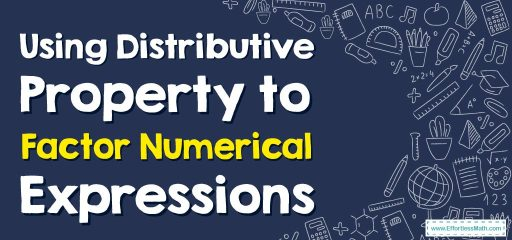# Using Distributive Property to Factor Numerical Expressions

In this article, the focus is on teaching you how to use the distributive property to factor numerical expressions. By understanding how to use the distributive property, you can simply factor variable expressions.## A step-by-step guide tousing the distributive property to factor numerical expressions

To factor numerical expressions, you have to find the greatest common factor (GCF) of the monomial terms.

To rewrite the polynomial as the product of the greatest common factor (GCF) and the additional parts of the polynomial, you have to use the distributive property.

“Distribute” means to divide something or give a part of something.

The rule of the distributive property is that multiplying the sum of two or more addends via a number equals multiplying each of the addends individually by the number and then adding the products together. Both of them give the same answer.
$$a(b+c)=a×b+a×c$$

### Using Distributive Property to Factor Numerical Expressions – Example 1

Use distributive property, factor the expression, $$40+25$$.
Solution:
Step 1: Find the greatest common factor of 40 and 25. It is 5.
Step 2: Divide each number by $$5. 40÷5=8, 25÷5=5.$$
Step 3: Use the distributive property to write an equivalent expression.
$$40+25=5×8+5×5=5(8+5)$$

Using Distributive Property to Factor Numerical Expressions – Example 2

Use distributive property, factor the expression, $$56+21$$.
Solution:
Step 1: Find the greatest common factor of 56 and 21. It is 7.
Step 2: Divide each number by $$7. 56÷7=8, 21÷7=3$$.
Step 3: Use the distributive property to write an equivalent expression.
$$56+21=7×8+7×3=7(8+3)$$

### What people say about "Using Distributive Property to Factor Numerical Expressions - Effortless Math: We Help Students Learn to LOVE Mathematics"?

No one replied yet.

X
30% OFF

Limited time only!

Save Over 30%

SAVE $5 It was$16.99 now it is \$11.99